# 类的六个默认成员函数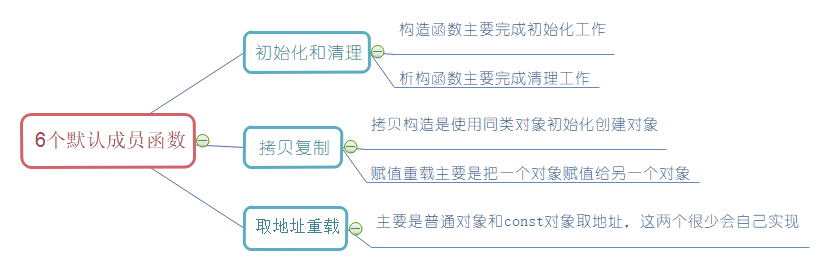# 构造函数和析构函数

## 1、构造

函数名与类名相同。 无返回值。 对象实例化时编译器自动调用对应的构造函数。 构造函数可以重载。

``````class Date
{
public:
//构造函数支持重载，那么也支持缺省（全缺省，半缺省，缺省）
Date(int year = 1, int month = 2, int day = 3)
{
_year = year;
_month = month;
_day = day;
}
void SetDate(int year, int month, int day)
{
_year = year;
_month = month;
_day = day;
}
void Display()
{
cout << _year << "-" << _month << "-" << _day << endl;
}
private:
int _year;
int _month;
int _day;
};
int main()
{
Date d1;
d1.SetDate(2018, 5, 1);
d1.Display();

Date d2(2021, 10, 21);
d2.Display();
//注意：如果通过无参构造函数创建对象时，对象后面不用跟括号，否则就成了函数声明
Date d3();
return 0;
}
``````

通过代码我们发现，当构造函数写成全缺省的时候，我们创建对象不传参也不会报错，编译器会把它当成默认构造函数（即无参），此时我们不需要在自己定义一个无参构造函数。 我们也可以在创建对象时传参，也不会报错，所以这是构造函数写成全缺省的好处 传参即有参构造，不传参即无参构造函数

这里需要注意的是，构造函数支持重载，我们通过有参构造函数去去初始化对象的时候，也要显示的写上无参构造函数，或者语法会出错。 如果类中没有显式定义构造函数，则C++编译器会自动生成一个无参的默认构造函数，一旦用户显式定义编译器将不再生成 构造函数既然可以重载，那么也支持缺省值的用法 无参的构造函数和全缺省的构造函数都称为默认构造函数，并且默认构造函数只能有一个。注意：无参构造函数、全缺省构造函数、我们没写编译器默认生成的构造函数，都可以认为是默认成员函数

``````class Time
{
public:
Time()
{
cout << "Time()" << endl;
_hour = 0;
_minute = 0;
_second = 0;
}
private:
int _hour;
int _minute;
int _second;
};
class Date
{
public:

private:
// 内置类型
int _year;
int _month;
int _day;
Time _tt;//自定义类型 struct、class、union
};
int main()
{
Date d;
return 0;
}
``````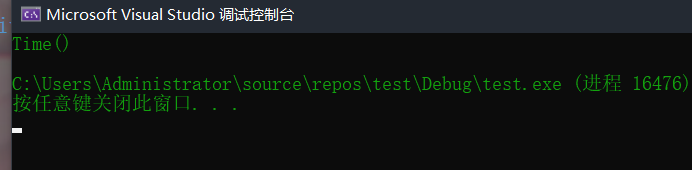C++把类型分成内置类型(基本类型)和自定义类型。内置类型就是语法已经定义好的类型：如int/char…，自定义类型就是我们使用class/struct/union自己定义的类型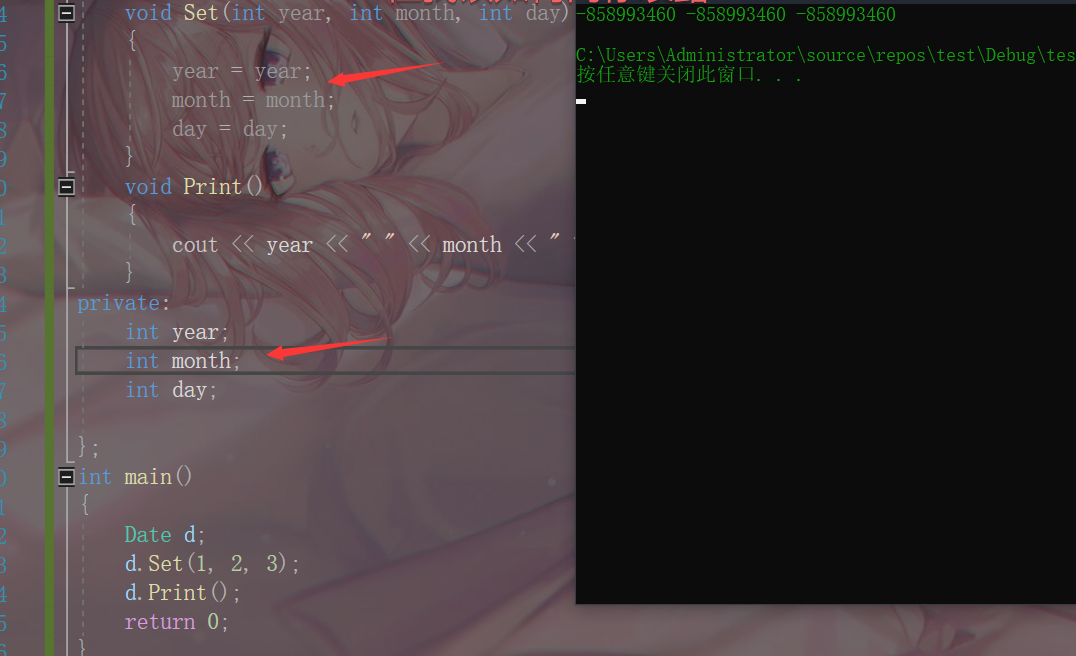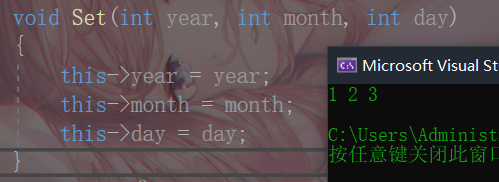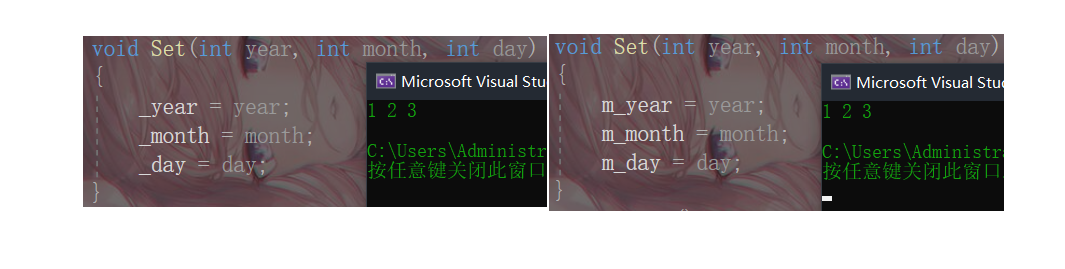## 2、析构

析构函数名是在类名前加上字符 ~。 无参数无返回值。 一个类有且只有一个析构函数。若未显式定义，系统会自动生成默认的析构函数。 对象生命周期结束时，C++编译系统系统自动调用析构函数
``````#include<iostream>
using namespace std;
#include<assert.h>
typedef int DataType;
class SeqList
{
public:
// 使用了缺省，当创建对象时不传参，也不会报错
SeqList(int capacity = 10)
{
_pData = (DataType*)malloc(capacity * sizeof(DataType));
assert(_pData);
_size = 0;
_capacity = capacity;
}
~SeqList()
{
if (_pData)
{
free(_pData); // 释放堆上的空间
_pData = NULL; // 将指针置为空
_capacity = 0;
_size = 0;
}
cout << "~SeqList()" << endl;
}
private:
int* _pData;
size_t _size;
size_t _capacity;
};
int main()
{
SeqList q(20);
//对象声明周期结束就会调用析构函数，清理指针_pData,_size,_capacity
return 0;
}
``````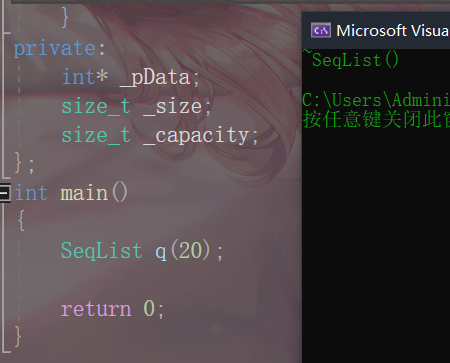``````class String
{
public:
String(const char* str = "jack")
{
_str = (char*)malloc(strlen(str) + 1);
strcpy(_str, str);
}
~String()
{
cout << "~String()" << endl;
free(_str);
}
private:
char* _str;
};
class Person
{
private:
String _name;
int _age;
};
int main()
{
Person p;
return 0;
}
``````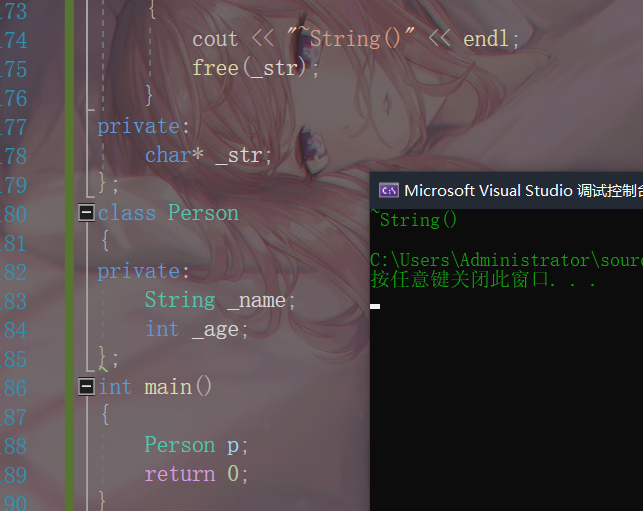## 3、拷贝构造函数

``````class Date
{
public:
Date(int year = 1900, int month = 1, int day = 1)
{
_year = year;
_month = month;
_day = day;
}
//拷贝构造函数也是构造函数，相当于重载
//const保证不修改，因为是拷贝，所以修改的话没有任何意义
//但这里必须得用引用，为什么？继续看下面
Date(const Date& d)
{
_year = d._year;
_month = d._month;
_day = d._day;
}
void Print()
{
cout << _year << " " << _month << " " << _day << endl;
}
private:
int _year;
int _month;
int _day;
};
int main()
{
Date d1;
Date d2(d1);//传参
d1.Print();
d2.Print();
return 0;
}
``````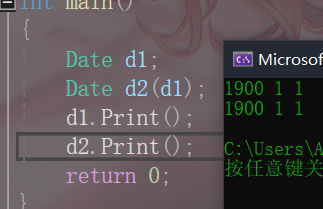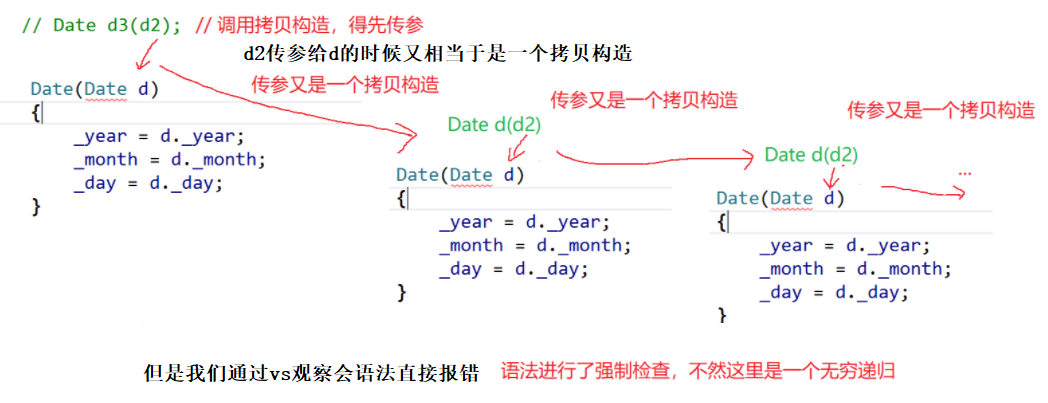``````class A
{
public:
A()
{

}
A(const A& a)
{
cout << "const A& a" << endl;
}
};
class Date
{
public:
Date(int year = 1900, int month = 1, int day = 1)
{
_year = year;
_month = month;
_day = day;
}
void Print()
{
cout << _year << " " << _month << " " << _day << endl;
}
private:
int _year;
int _month;
int _day;
A _a;
};
int main()
{
Date d1;
// 这里d2调用的默认拷贝构造完成拷贝，d2和d1的值也是一样的
Date d2(d1);
d1.Print();
d2.Print();
return 0;
}
``````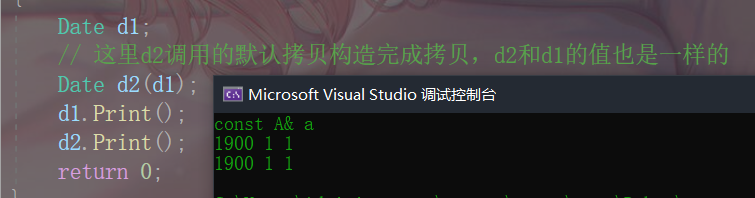1、内置类型，字节序的浅拷贝（按字节序的拷贝）
2、自定义类型，会去调用他的拷贝构造完成拷贝

``````class String
{
public:
String(const char* str = "jack")
{
_str = (char*)malloc(strlen(str) + 1);
strcpy(_str, str);
}
~String()
{
cout << "~String()" << endl;
free(_str);
}
private:
char* _str;
};
int main()
{
String s1("hello");
String s2(s1);
}
``````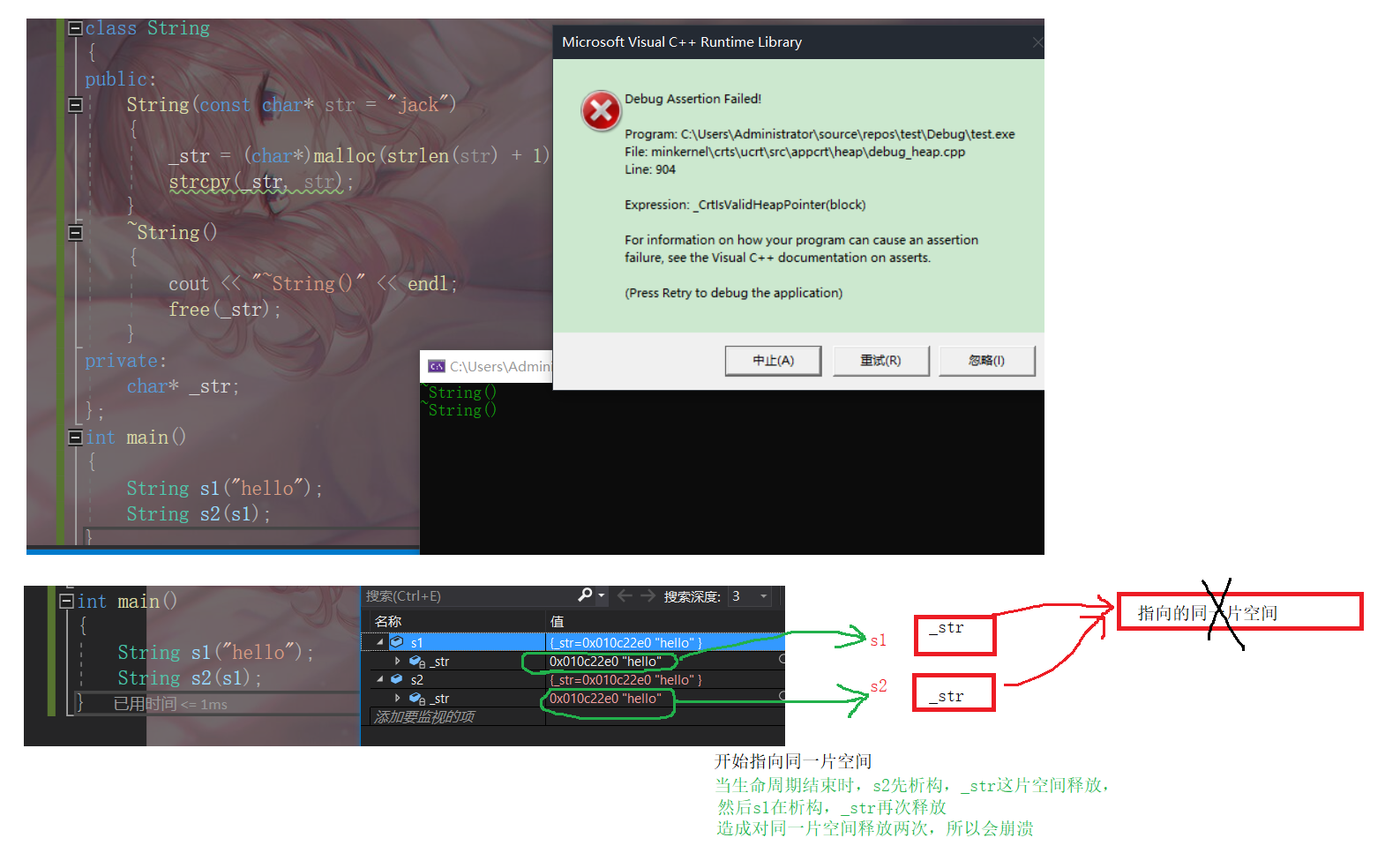拷贝构造函数是构造函数的一个重载形式。 拷贝构造函数的参数只有一个且必须使用引用传参，使用传值方式会引发无穷递归调用 要注意浅拷贝带来的危险

# 赋值运算符重载

``````class Date
{
public:
Date(int year = 1900, int month = 1, int day = 1)
{
_year = year;
_month = month;
_day = day;
}
//private:
int _year;
int _month;
int _day;
};
// 这里会发现运算符重载成全局的就需要成员变量是共有的，那么问题来了，封装性如何保证？
// 这里其实可以用我们后面学习的友元解决，或者干脆重载成成员函数。
bool operator==(const Date& d1, const Date& d2)
{
return d1._year == d2._year
&& d1._month == d2._month
&& d1._day == d2._day;
}
void Test()
{
Date d1(2018, 9, 26);
Date d2(2018, 9, 27);
cout << (d1 == d2) << endl;
}
int main()
{
Test();
return 0;
}
``````

``````class Date
{
public:
Date(int year = 1900, int month = 1, int day = 1)
{
_year = year;
_month = month;
_day = day;
}
bool operator==(const Date& d2)
{
return _year == d2._year
&& _month == d2._month
&& _day == d2._day;
}
//private:
int _year;
int _month;
int _day;
};
int main()
{
Date d1(2018, 9, 26);
Date d2(2018, 9, 27);
d1 == d2;
// d1==d2 -> d1.operator(d2) -> d1.operator(&d1,d2)
d1.operator==(d2);// 与d1==d2效果一样
return 0;
}
``````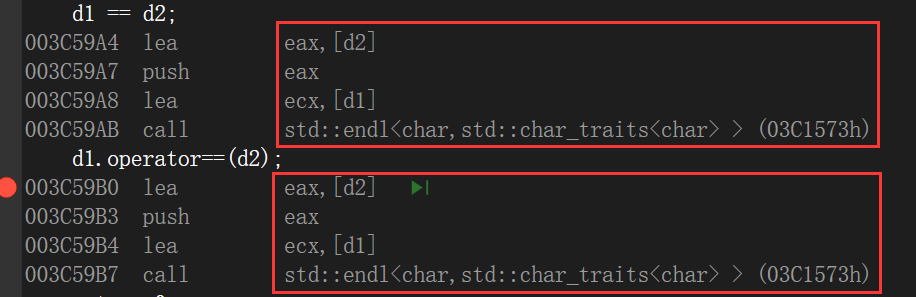``````class Date
{
public:
Date(int year = 1900, int month = 1, int day = 1)
{
_year = year;
_month = month;
_day = day;
}
Date(const Date& d)
{
_year = d._year;
_month = d._month;
_day = d._day;
}
Date& operator=(const Date& d)
{
if (this != &d)
{
_year = d._year;
_month = d._month;
_day = d._day;
}
return *this;
//注意返回引用，为什么就不用我说了，前面讲到的临时变量
}
void Traverse()
{
cout << _year << _month << _day << endl;
}
private:
int _year;
int _month;
int _day;
};
int main()
{
Date d1(2021, 10, 22);
Date d2;
d2 = d1;
d2.Traverse();
return 0;
}
``````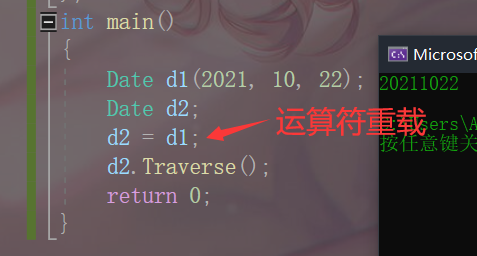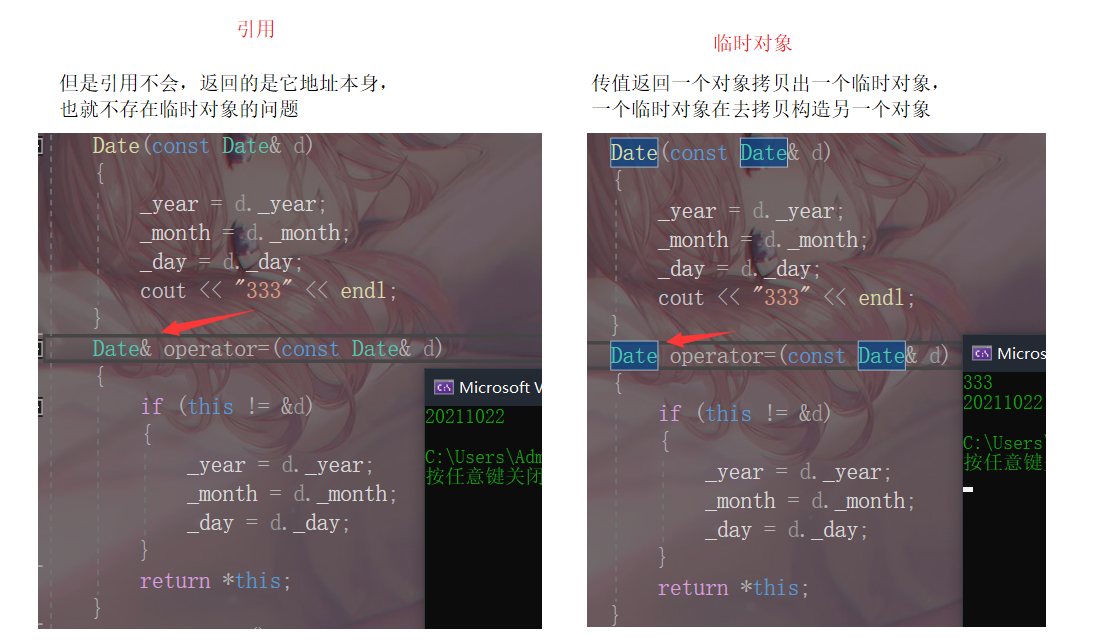参数类型 返回值 检测是否自己给自己赋值 返回*this 一个类如果没有显式定义赋值运算符重载，编译器也会生成一个，完成对象按字节序的值拷贝

运算符默认都是给内置类型变量使用的 自定义类型的变量想用这些运算符，得自己进行运算符重载 自己进行运算符重载，也就是写一个函数去定义运算符的行为

# const成员

const修饰类的成员函数：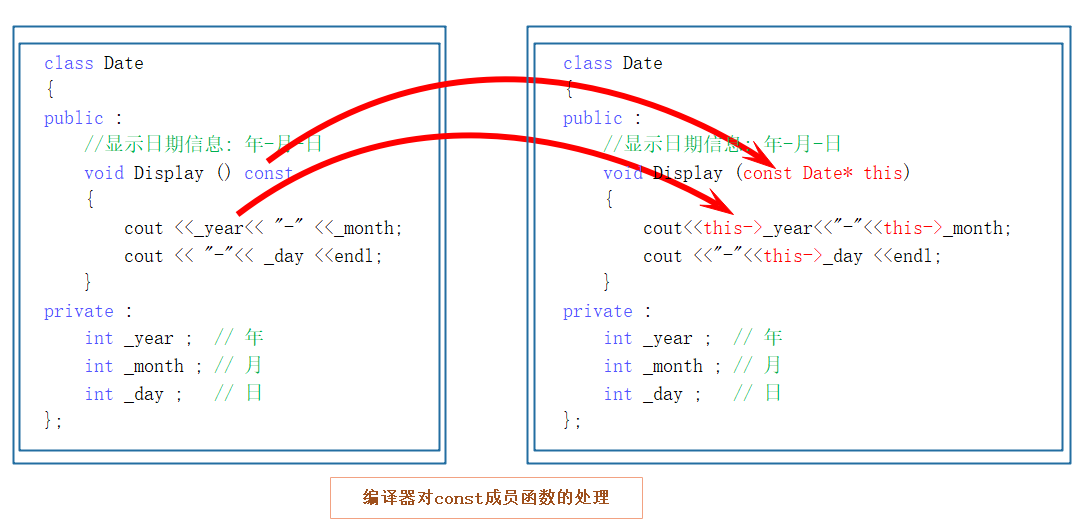``````class Date
{
public:
//加了const，表示成员函数为一个常函数，不能对任何成员做修改
void Display() const
{
cout << _year << " " << _month << " " << _day << endl;
}
private:
int _year = 0; // 年
int _month = 0; // 月
int _day = 0; // 日
};
void Test()
{
Date d1;
d1.Display();

}
int main()
{
Test();
return 0;
}
``````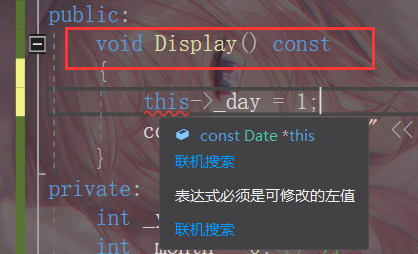const总结

成员函数加const，是有好处的，这样const 对象 和 非const对象都可以调用 不是所有成员函数都要加const 如果一个成员函数内部需要修改成员变量，那就不用加const，比如初始化的函数等 如果对成员不做任何修改的函数，在写的时候最好加上const

const 常考

const对象可以调用非const成员函数吗？ 不能 非const对象可以调用const成员函数吗？ 能 const成员函数内可以调用其它的非const成员函数吗？ 不能 非const成员函数内可以调用其它的const成员函数吗？ 能

const权限可以缩小，不能方法